# Chapter 12 High-level genomic functions

In this chapter, several high-level functions which create tracks are introduced.

## 12.1 Ideograms

circos.initializeWithIdeogram() initializes the circular plot and adds ideogram track if the cytoband data is available. Actually, the ideograms are drawn by circos.genomicIdeogram(). circos.genomicIdeogram() creates a small track of ideograms and can be used anywhere in the circle. By default it adds ideograms for human genome hg19 (Figure 12.1).

circos.initializeWithIdeogram(plotType = c("labels", "axis"))
circos.track(ylim = c(0, 1))
circos.genomicIdeogram() # put ideogram as the third track
circos.genomicIdeogram(track.height = 0.2)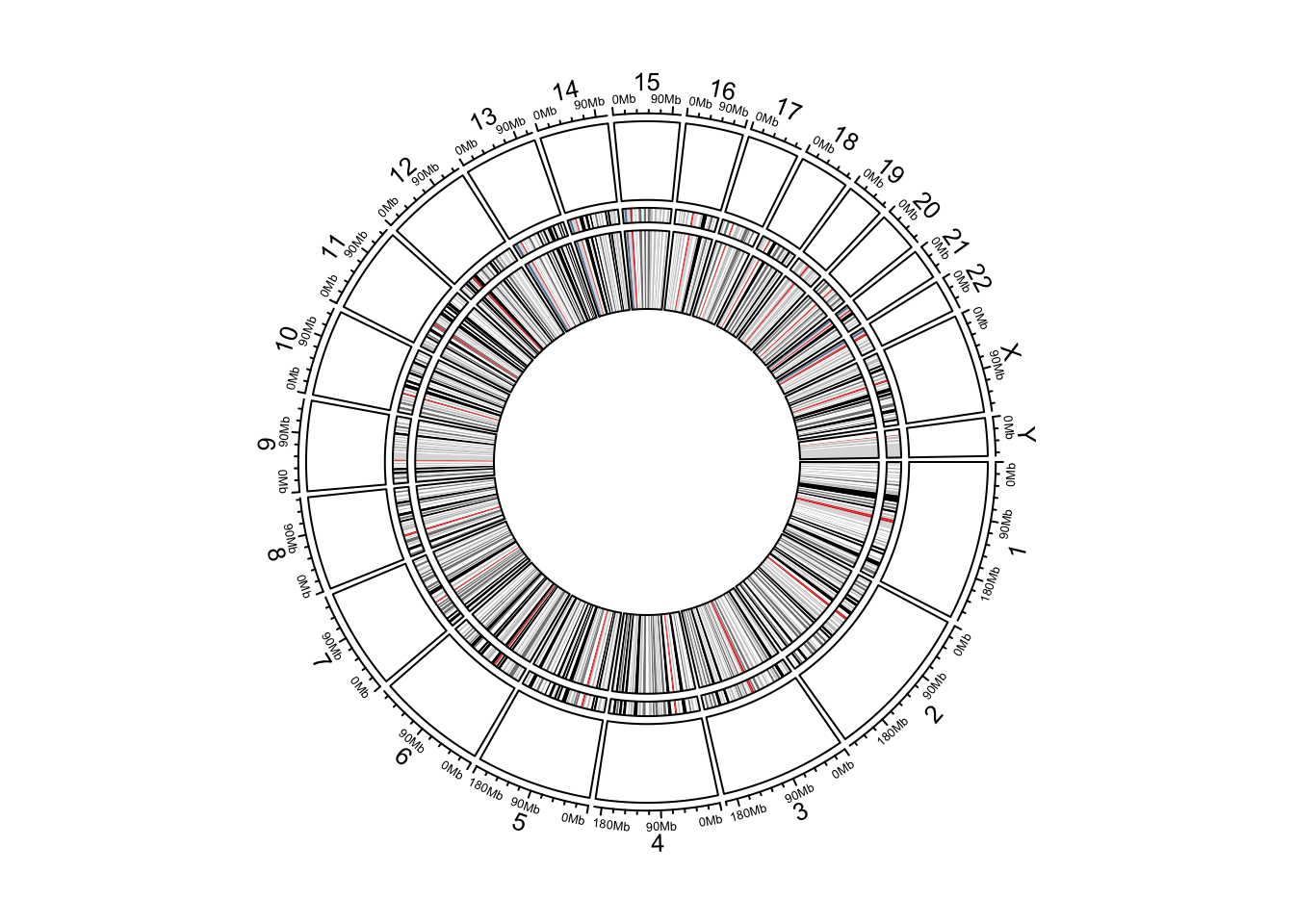Figure 12.1: Circular ideograms.

## 12.2 Heatmaps

Matrix which corresponds to genomic regions can be visualized as heatmaps. Heatmaps completely fill the track and there are connection lines connecting heatmaps and original positions in the genome. circos.genomicHeatmap() draws connection lines and heatmaps as two tracks and combines them as an integrated track.

Generally, all numeric columns (excluding the first three columns) in the input data frame are used to make the heatmap. Columns can also be specified by numeric.column which is either an numeric vector or a character vector. Colors can be specfied as a color matrix or a color mapping function generated by colorRamp2().

The height of the connection line track and the heatmap track can be set by connection_height and heatmap_height arguments. Also parameters for the styles of lines and rectangle borders can be adjusted, please check the help page of circos.genomicHeatmap().

circos.initializeWithIdeogram()
bed = generateRandomBed(nr = 100, nc = 4)
col_fun = colorRamp2(c(-1, 0, 1), c("green", "black", "red"))
circos.genomicHeatmap(bed, col = col_fun, side = "inside", border = "white")
circos.clear()

In the left figure in Figure 12.2, the heatmaps are put inside the normal genomic track. Heatmaps are also be put outside the normal genomic track by setting side = "outside" (Figure 12.2, right).

circos.initializeWithIdeogram(plotType = NULL)
circos.genomicHeatmap(bed, col = col_fun, side = "outside",
line_col = as.numeric(factor(bed[])))
circos.genomicIdeogram()
circos.clear()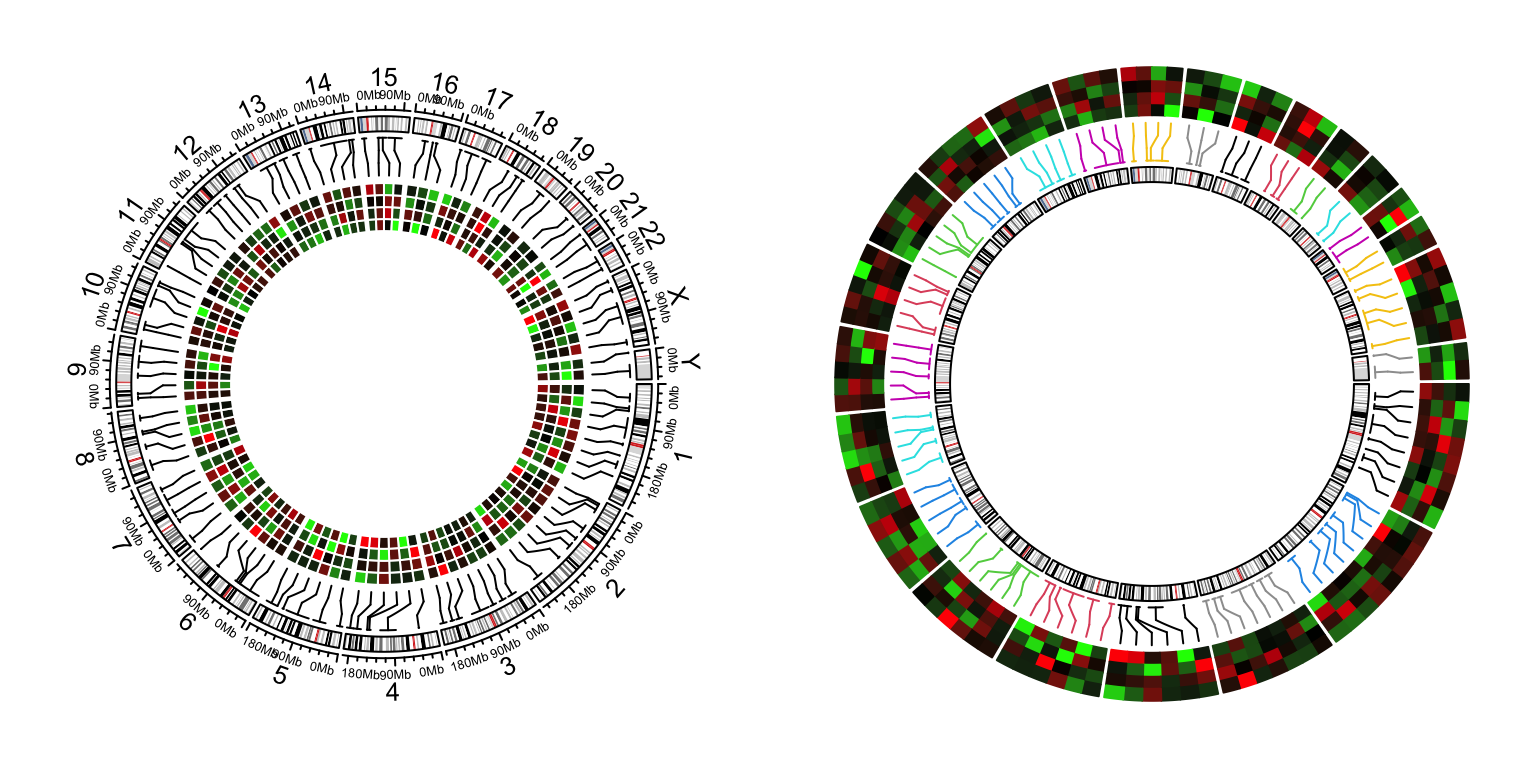Figure 12.2: Genomic heamtaps.

## 12.3 Labels

circos.genomicLabels() adds text labels for regions that are specified. Positions of labels are automatically adjusted so that they do not overlap to each other.

Similar as circos.genomicHeatmap(), circos.genomicLabels() also creates two tracks where one for the connection lines and one for the labels. You can set the height of the labels track to be the maximum width of labels by labels_height = max(strwidth(labels)). padding argument controls the gap between two neighbouring labels.

circos.initializeWithIdeogram()
bed = generateRandomBed(nr = 50, fun = function(k) sample(letters, k, replace = TRUE))
bed[1, 4] = "aaaaa"
circos.genomicLabels(bed, labels.column = 4, side = "inside")
circos.clear()

Similarlly, labels can be put outside of the normal genomic track (Figure 12.3 right).

circos.initializeWithIdeogram(plotType = NULL)
circos.genomicLabels(bed, labels.column = 4, side = "outside",
col = as.numeric(factor(bed[])), line_col = as.numeric(factor(bed[])))
circos.genomicIdeogram()
circos.clear()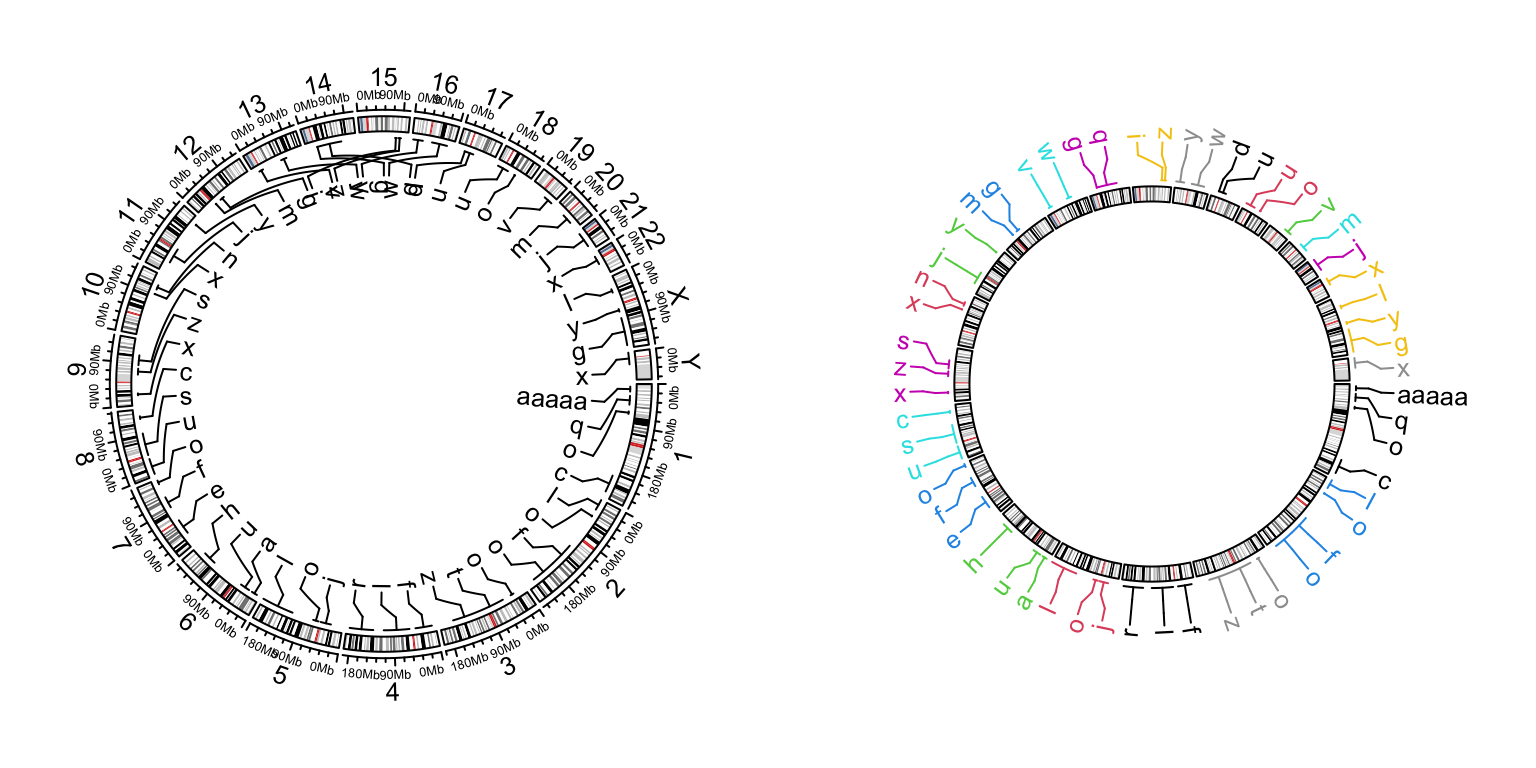Figure 12.3: Genomic labels.

## 12.4 Genomic axes

The genomic axes are not really high-level graphics, but it is better to also introduce here. For circos.initializeWithIdeogram(), by default it draws axes with tick labels properly formatted. The axes are internally implemented by circos.genomicAxis() and it can be used to add genomic axes at any track (Figure 12.4).

circos.initializeWithIdeogram(plotType = NULL)
circos.genomicIdeogram()
# still work on the ideogram track
circos.track(track.index = get.current.track.index(), panel.fun = function(x, y) {
circos.genomicAxis(h = "top")
})
circos.track(ylim = c(0, 1), track.height = 0.1)
circos.track(track.index = get.current.track.index(), panel.fun = function(x, y) {
circos.genomicAxis(h = "bottom", direction = "inside")
})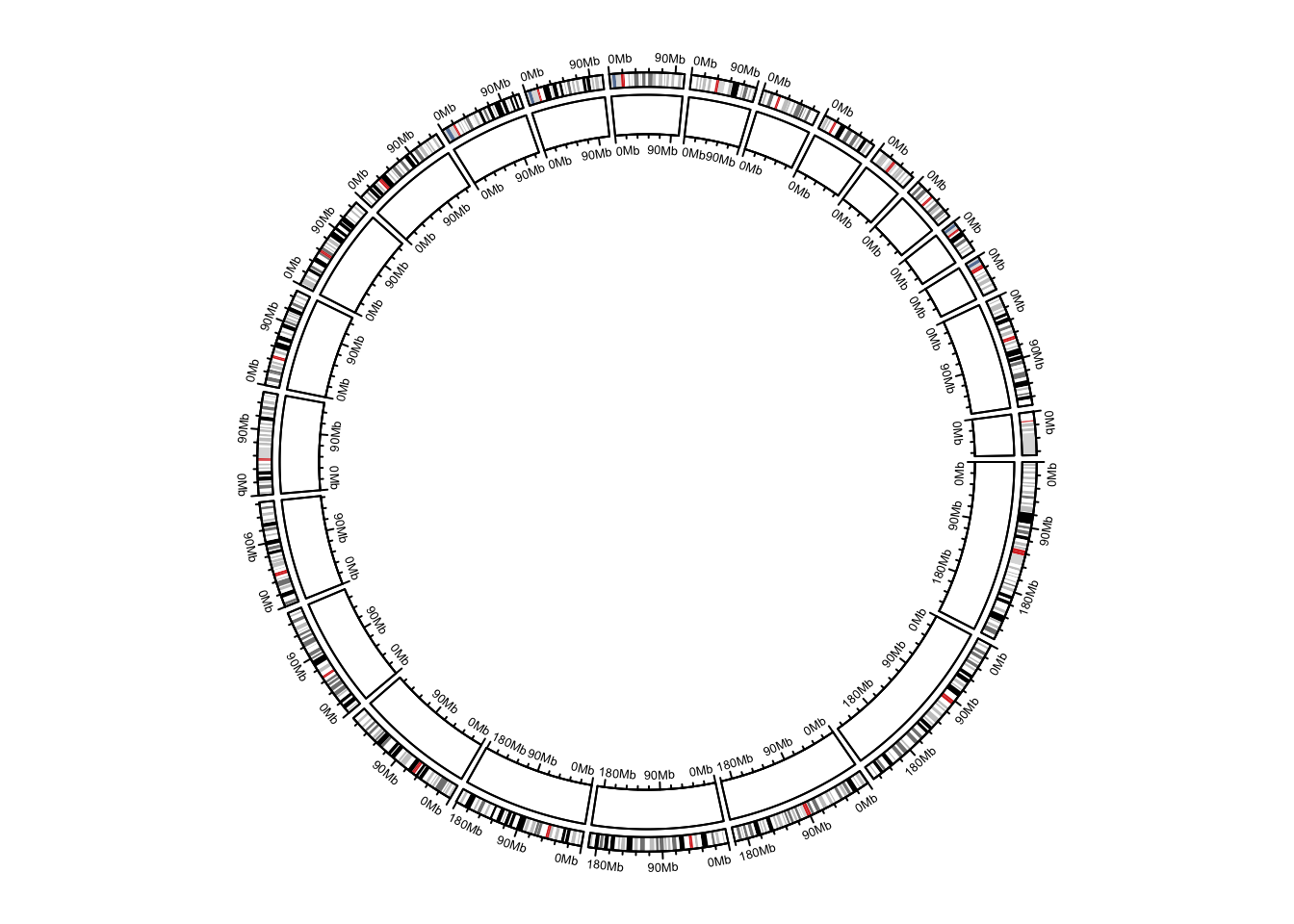circos.clear()

## 12.5 Genomic density and Rainfall plot

Rainfall plots are used to visualize the distribution of genomic regions in the genome. Rainfall plots are particularly useful to identify clusters of regions. In the rainfall plot, each dot represents a region. The x-axis corresponds to the genomic coordinate, and the y-axis corresponds to the minimal distance (log10 transformed) of the region to its two neighbouring regions. A cluster of regions will appear as a “rainfall” in the plot.

circos.genomicRainfall() calculates neighbouring distance for each region and draw as points on the plot. Since circos.genomicRainfall() generates data on y-direction (log10(distance)), it is actually a high-level function which creates a new track.

The input data can be a single data frame or a list of data frames.

circos.genoimcRainfall(bed)
circos.genoimcRainfall(bed_list, col = c("red", "green"))

However, if the amount of regions in a cluster is high, dots will overlap, and direct assessment of the number and density of regions in the cluster will be impossible. To overcome this limitation, additional tracks are added which visualize the genomic density of regions (defined as the fraction of a genomic window that is covered by genomic regions).

circos.genomicDensity() calculates how much a genomic window is covered by regions in bed. It is also a high-level function and creates a new track.

The input data can be a single data frame or a list of data frames.

circos.genomicDensity(bed)
circos.genomicDensity(bed, baseline = 0)
circos.genomicDensity(bed, window.size = 1e6)
circos.genomicDensity(bedlist, col = c("#FF000080", "#0000FF80"))

Following example makes a rainfall plot for differentially methylated regions (DMR) and their genomic densities. In the plot, red corresponds to hyper-methylated DMRs (gain of methylation) and blue corresponds to hypo-methylated DMRs (loss of methylation). You may see how the combination of rainfall track and genomic density track helps to get a more precise inference of the distribution of DMRs on genome (Figure 12.5).

load(system.file(package = "circlize", "extdata", "DMR.RData"))
circos.initializeWithIdeogram(chromosome.index = paste0("chr", 1:22))

bed_list = list(DMR_hyper, DMR_hypo)
circos.genomicRainfall(bed_list, pch = 16, cex = 0.4, col = c("#FF000080", "#0000FF80"))
circos.genomicDensity(DMR_hyper, col = c("#FF000080"), track.height = 0.1)
circos.genomicDensity(DMR_hypo, col = c("#0000FF80"), track.height = 0.1)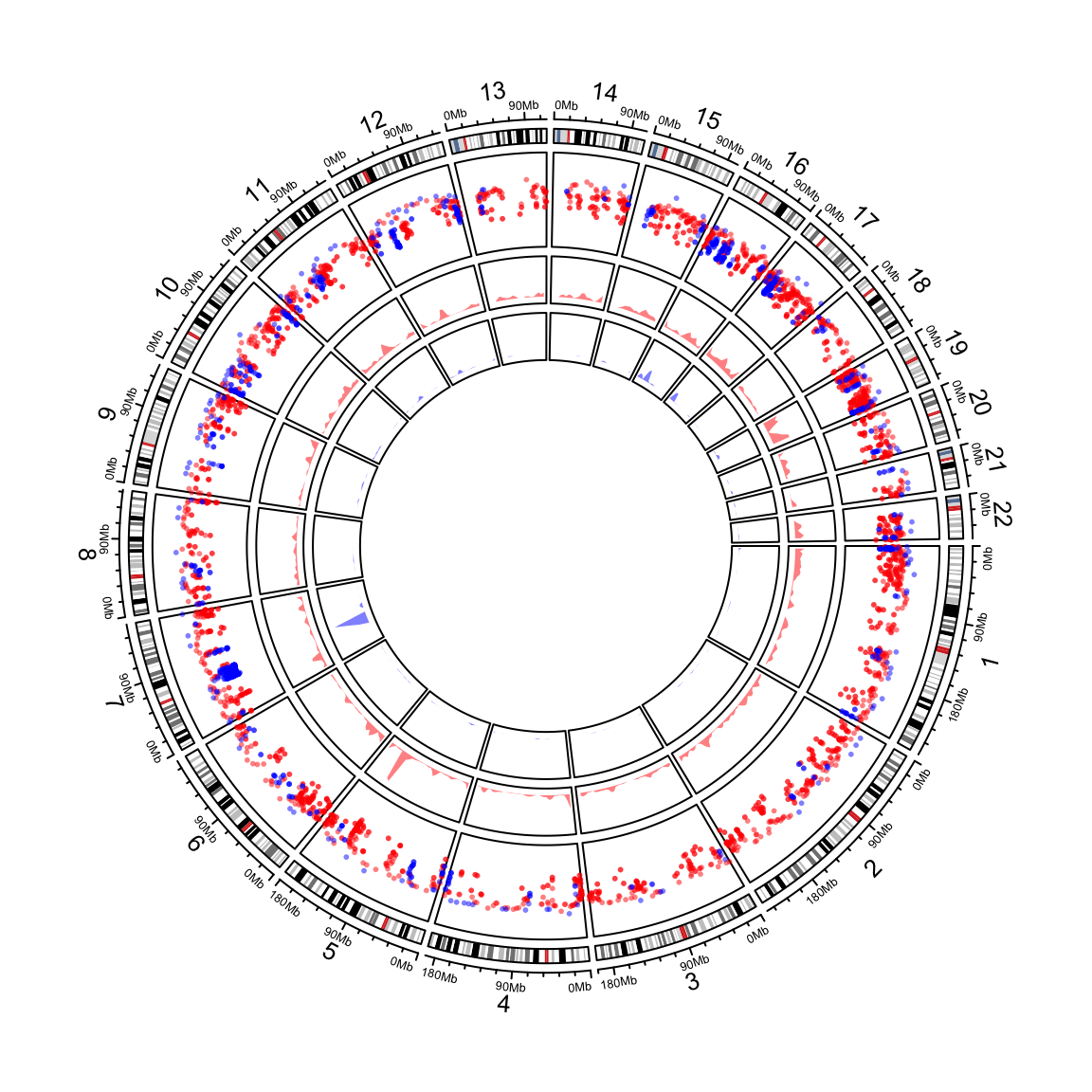Figure 12.5: Genomic rainfall plot and densities.

circos.clear()

circos.genomicDensity() also supports to calculate the overlap as the number of the regions that overlap to each window by setting count_by = "number".

circos.initializeWithIdeogram(chromosome.index = paste0("chr", 1:22))
circos.genomicDensity(DMR_hyper, col = c("#FF000080"), track.height = 0.1)
circos.genomicDensity(DMR_hyper, col = c("#FF000080"), count_by = "number", track.height = 0.1)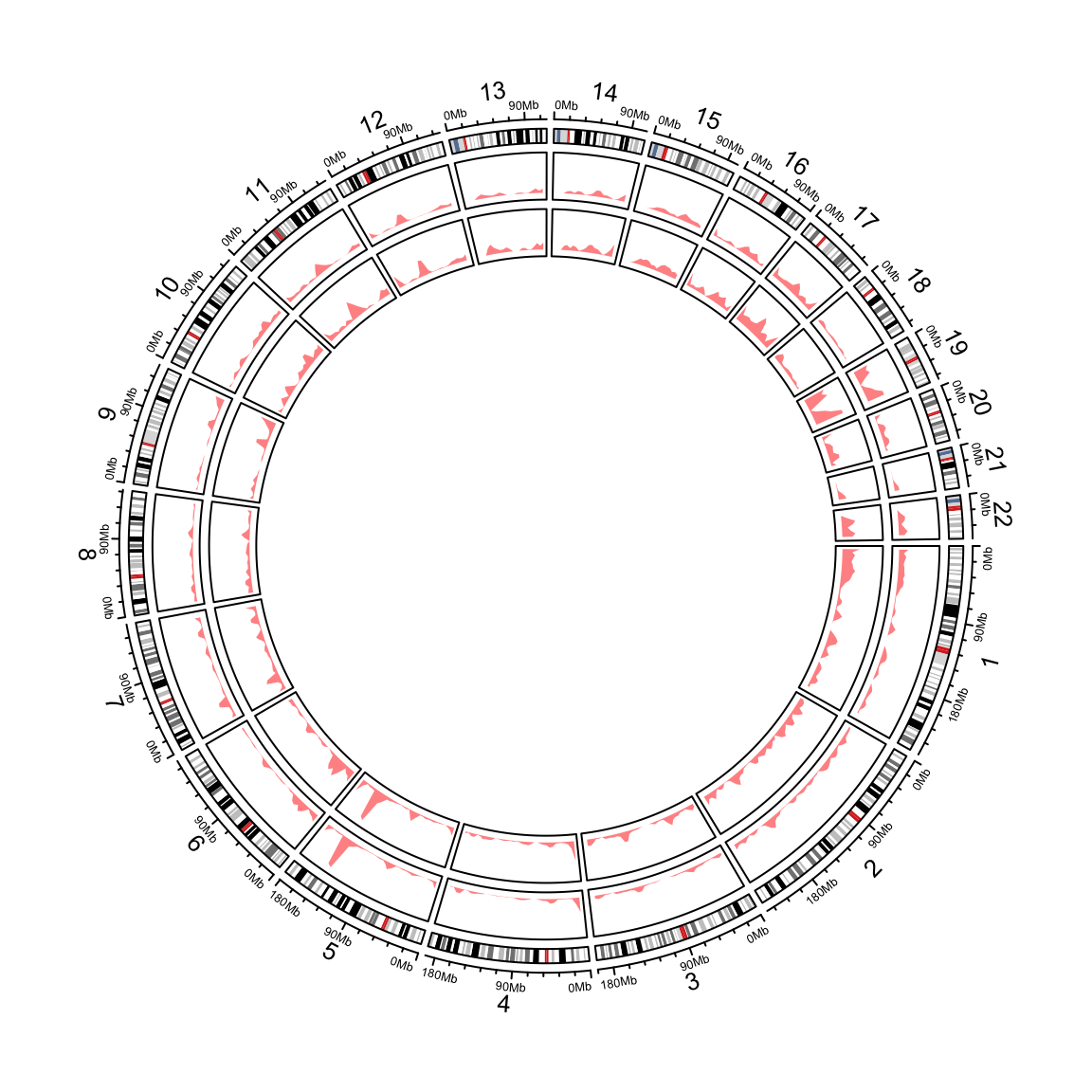Figure 12.6: Genomic densities.

circos.clear()

Internally, rainfallTransform() and genomicDensity() are used to the neighbrouing distance and the genomic density values.

head(rainfallTransform(DMR_hyper))
##      chr   start     end  dist
## 70  chr1  933445  934443 35323
## 104 chr1  969766  970362  4909
## 105 chr1  975271  976767  4909
## 154 chr1 1108819 1109923 31522
## 155 chr1 1141445 1142405 31522
## 157 chr1 1181550 1182782 39145
head(genomicDensity(DMR_hyper, window.size = 1e6))
##    chr   start     end    value
## 1 chr1       1 1000000 0.003093
## 2 chr1  500001 1500000 0.007592
## 3 chr1 1000001 2000000 0.008848
## 4 chr1 1500001 2500000 0.010155
## 5 chr1 2000001 3000000 0.011674
## 6 chr1 2500001 3500000 0.007783